# NEET Physics Wave Optics Questions Solved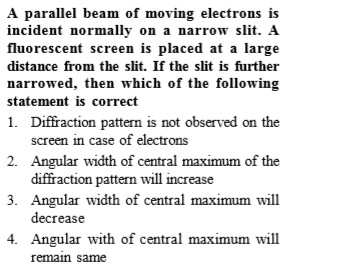Complete Question Bank + Test Series
Complete Question Bank

Difficulty Level: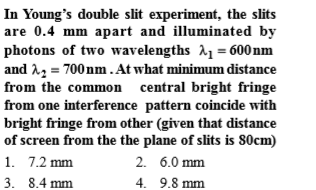Complete Question Bank + Test Series
Complete Question Bank

Difficulty Level: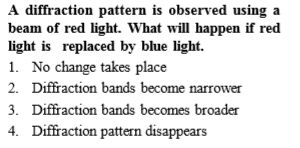Complete Question Bank + Test Series
Complete Question Bank

Difficulty Level: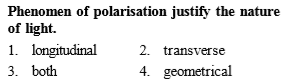Complete Question Bank + Test Series
Complete Question Bank

Difficulty Level: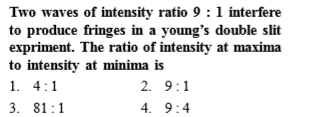Complete Question Bank + Test Series
Complete Question Bank

Difficulty Level: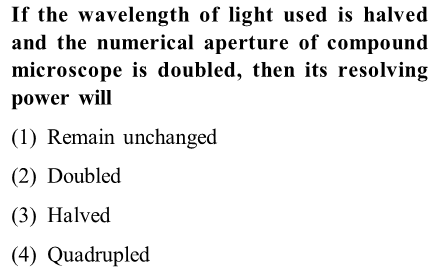Complete Question Bank + Test Series
Complete Question Bank

Difficulty Level: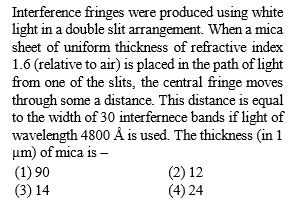Complete Question Bank + Test Series
Complete Question Bank

Difficulty Level:

Young's double slit experiment is first performed in air and then in a medium other than air. It is found that 8th bright fringe in the medium lies where 5th dark fringe lies in air. The refractive index of the medium is nearly

(a)1.25

(b) 1.59

(c) 1.69

(d) 1.78

Complete Question Bank + Test Series
Complete Question Bank

Difficulty Level:

Two polarids  are placed with their axis perpendicular to each other. Unpolarised light ${I}_{o}$ is incident on ${P}_{1}$. A third polaroid ${P}_{3}$ is kept in between  such that its axis makes an angle ${45}^{°}$ with that of ${P}_{1}$. The intensity of transmitted light through ${P}_{2}$

(a)$\frac{{I}_{o}}{2}$

(b) $\frac{{I}_{o}}{4}$

(c) $\frac{{I}_{o}}{8}$

(d) $\frac{{I}_{o}}{16}$

Complete Question Bank + Test Series
Complete Question Bank

Difficulty Level:

The interference pattern is obtained with two coherent light sources of intensity ratio n. In the interference pattern, the ratio $\frac{{I}_{max}-{I}_{min}}{{I}_{max}+{I}_{min}}$ will be

(a) $\frac{\sqrt{n}}{n+1}$             (b) $\frac{2\sqrt{n}}{n+1}$

(c) $\frac{\sqrt{n}}{{\left(n+1\right)}^{2}}$         (d) $\frac{2\sqrt{n}}{{\left(n+1\right)}^{2}}$